# The Scheme 13:9 (parser)

Warning

This page is referring to child section which source is inherited by the prime spin with partitions listed below. Please return always to the Home Page to track the hierarchy.

This recombination is taking a scheme called DNA Recombination: M+F to C1+C2 which could only be happen when two (2) chromosomes involve. Here we found that each of their DNA will form a scheme called The Scheme 13:9 as shown below.

``````===========
(1){1}-7:   7'
(1){8}-13:  6‘
(1)14-{19}: 6‘
------------- {6+6} --→ [M+F]
(2)20-24:   5'            |
(2)25-{29}: 5'            |
------------  5+5 --------
(3)30-36:   7:{70,30,10²} |
------------              |
(4)37-48:   12• ---       |
(5)49-59:   11°    |      |
--}30° 30•    |
(6)60-78:   19°    |      |
(7)79-96:   18• ---       |
--------------            |
(8)97-109:  13            |
(9)110-139:{30}=5x6 <--x-- [C1+C2]
--
43
``````

There will be two (2) schemes are generated from Scheme-12 which are Scheme-53 and Scheme-34. The Scheme-53 is initially build using 12 kind of 12 concecutife primes that is laid in sequence. Here we are gonna use 12 repositories to simulate it.

``````True Prime Pairs:
(5,7), (11,13), (17,19)

|------------------------ Scheme-12 ------------------------|
|------------ 6¤ -------------|------------- 6¤ ------------|
|--------------------------- 192 ---------------------------|
|---- {23} ----|---- {49} ----|-- {29} -|--{30} --|-- 61 ---|
+----+----+----+----+----+----+----+----+----+----+----+----+
|  5 |  7 | 11 |{13}| 17 | 19 | 17 |{12}| 11 | 19 | 18 | 43 |
+----+----+----+----+----+----+----+----+----+----+----+----+
|---------  5¤  ---------|---- {48} ----|----- {48} ---|{43}|
|---------  5¤  ---------|------------ {96} -----------|{43}|
|--------- {53} ---------|-------------- {139} -------------|
|------ Scheme-53 -------|------------ Scheme-34 -----------|
``````

From the primes flatform we got 109 objects including for the 7 rows. So the encapsulation goes to the rest of 102. By the next level this 102 will represent 100 of 60 and 40 nodes of previous 619 primes of 2nd and 3rd layers in form of (2,60,40).

168 + 329 + 289 - 619 - 30 - 30 - 5 = 786 - 619 - 65 = 102 = (2,60,40)

The 10 ranks will coordinate with the 18 to raise up the symmetrical behaviour of 12+24=36 which is prime pair 17+19=36 and let the 2 and 3 out of 2,3,5,7 to begin a new cycle while the 5,7 will pair the 11,13 and 17,19 as True Prime Pairs.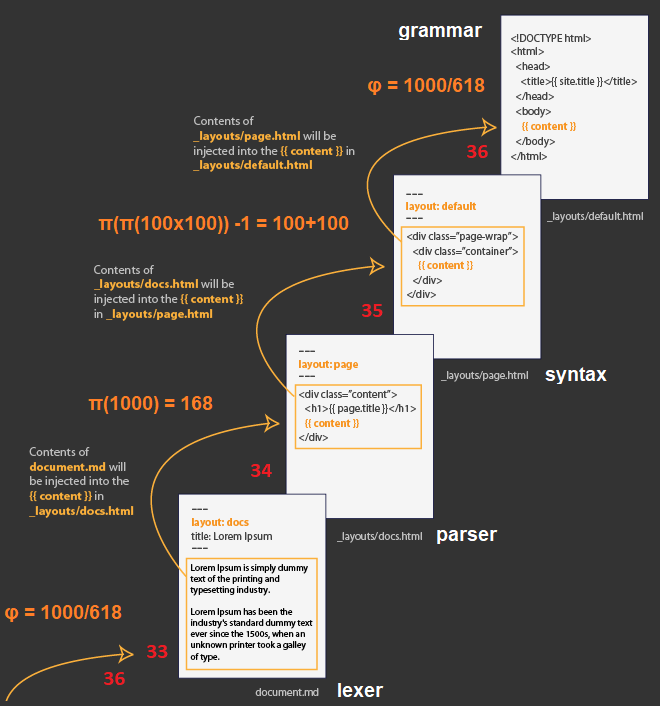By having the total of 168, the 102 and the 30+36=66 will take the 1st and 2nd prime on The Primes Platform. This leads to 168 - 29 - 96 = 139 - 96 = 43 primes on the last of 7th row. That what and why 18+13+12=43 by the last 9 cells is standing for!

``````  -----------------+----+----+----+----+----+----+----+----+----+-----
The last 9 cells |  1 |  2 |  3 |  4 |  5 |  6 |  7 |  8 |  9 | Sum
=================+====+====+====+====+====+====+====+====+====+=====
3,2→18+13+12→43  | 73 | 79 | 83 | 89 | 97 | 101| 103| 107| 109|29th →13th→ 2,3
-----------------+----+----+----+----+----+----+----+----+----+-----

- This 43 is 18+13+12 in bilateral on perfect square of 9 goes to 89 → π(89²) = 1000
``````

This 71 is a conformation that it has the same result as we have explained on the residual objects of 571 turn to a vektor of 71 while the rest of 500 turn to 200 objects of 3's identity and the last objects of 300 goes to the next cycles.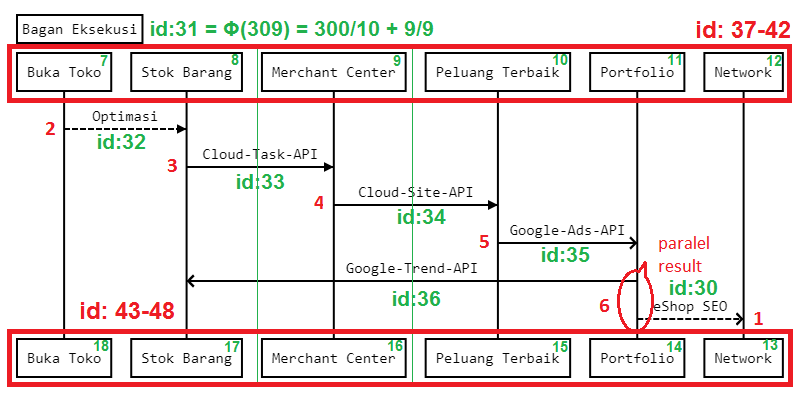So now out of 1000 numbers that generated from 1000 primes we will get the rest of 1000 - 100 = 900. This 900 will behave as matrix square 30x30 and act as the base frame of 2nd and 3rd layer which are working on π(π(100x100))-1=200 primes:

``````Scheme:
168 + 329 + 289 = 786
d(786) = d(7+8+6) = d(21) = d(3)
10+29+109+(109-100)²+100x10=π(10000)

Modulus:
30  «   60  »  90
|       |       |
3:29 « 1:6:8 » 28:9
└── 3 + └── 6  + └── 9 = 18

|       2'     |        3'         |              5'              |               7'             |
|--------------|-------------------|------------------------------|------------------------------|
|     lexering = π(1000)           |                    parsering = 1000/Φ                       |
|--------------|-------------------|------------------------------|------------------------------|
|   mapping    |     feeding       |          syntaxing           |          grammaring          |
+----+----+----+----+----+----+----+----+----+-----+----+----+----+----+----+-----+----+----+----+
| 30 | 31 | 32 | 33 | 34 | 35 | 36 | 37 | 38 |  39 | 40 | 41 | 42 | 43 | 44 | 45  | 46 | 47 | 48 |
+----+----+----+----+----+----+----+----+----+-----+----+----+----+----+----+-----+----+----+----+
|  2 | 60 | 40 | 1  | 30 | 30 | 5  | 1  | 30 | 200 |  8 | 40 | 50 |  1 | 30 | 200 |  8 | 10 | 40 |
+----+----+----+----+----+----+----+----+----+-----+----+----+----+----+----+-----+----+----+----+
|  ° |ΔΔΔΔ  ΦΦ | •    ΔΔ   ΔΔ   ¤  | •    ΔΔ   ΦΦΦ    Φ   ΦΦ  ¤¤¤¤|  •   ΔΔ   ΦΦΦ    Φ   ¤¤   ΦΦ |

|----- 102  ---|-------  66  ------|-------- 329 = 7 x 47 --------|- 289 = (8+9)² = 2 & (2³+9²) -|
|---2x3x(8+9)--|---- 2x3x(2+9) ----|---- (1+2) & (2x9)+(2+9) -----|------ 2 & (8x9)+(8+9) -------|
|--------- 168 = π(1000) ----------|------ 1229 = π(10000) -------|------ π(89²) = 1000 ---------|
|--------- 168 = π(618xΦ) ---------|--------------- 618 = 1000/Φ = 1000x1000/1618 ---------------|

``````

This polarity is happened per six (6) cycles by the polar of six (6) to one (1) and six (6) to seven (7) by which we finally found if this behaviour is cascaded bilaterally within the correlation between 61 as the 18th prime and 67 as the 19th prime.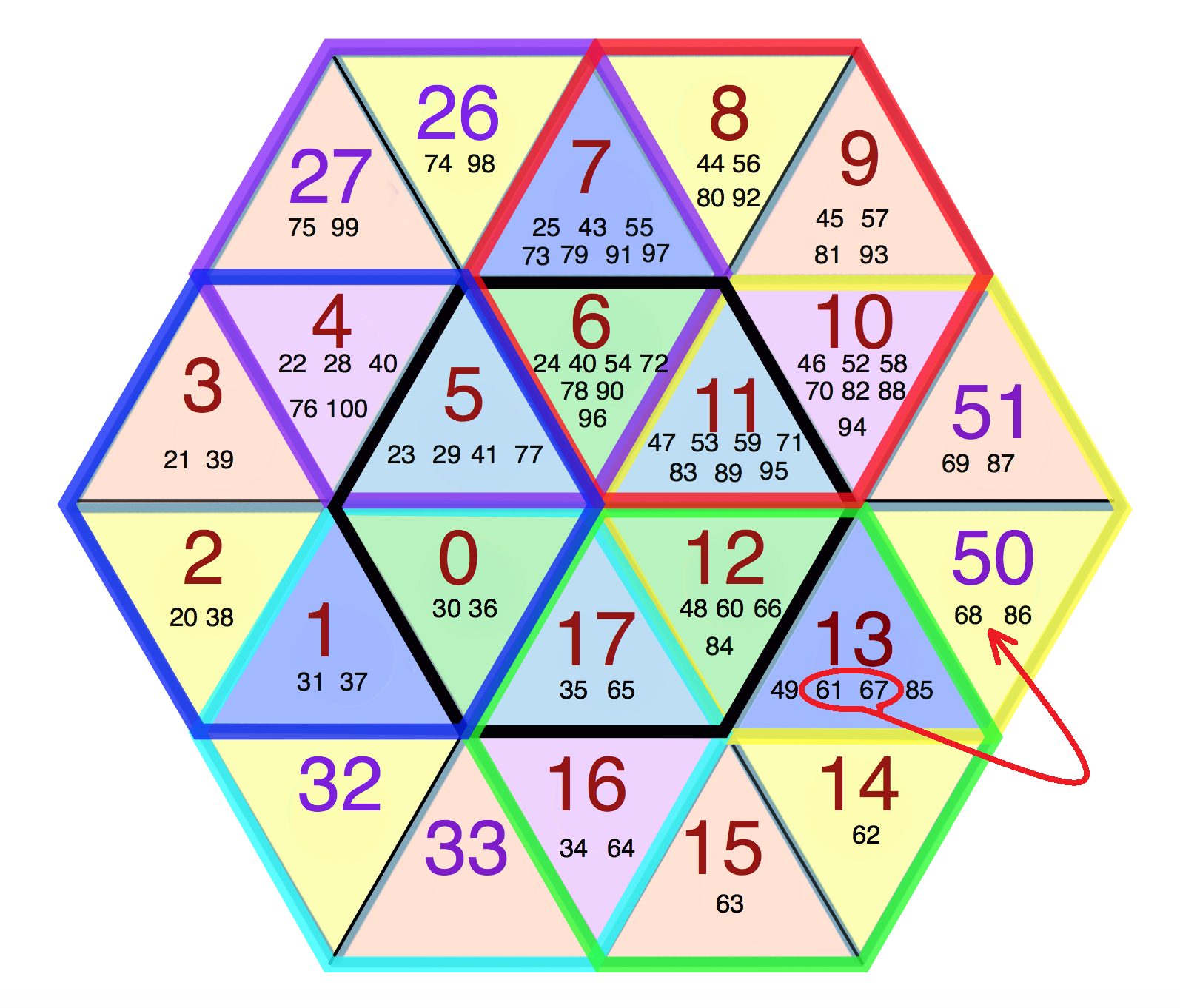The Parser which takes the tokens from the lexer and returns a syntax tree based on a grammar. The grammar is the language of languages and provides the rules and syntax.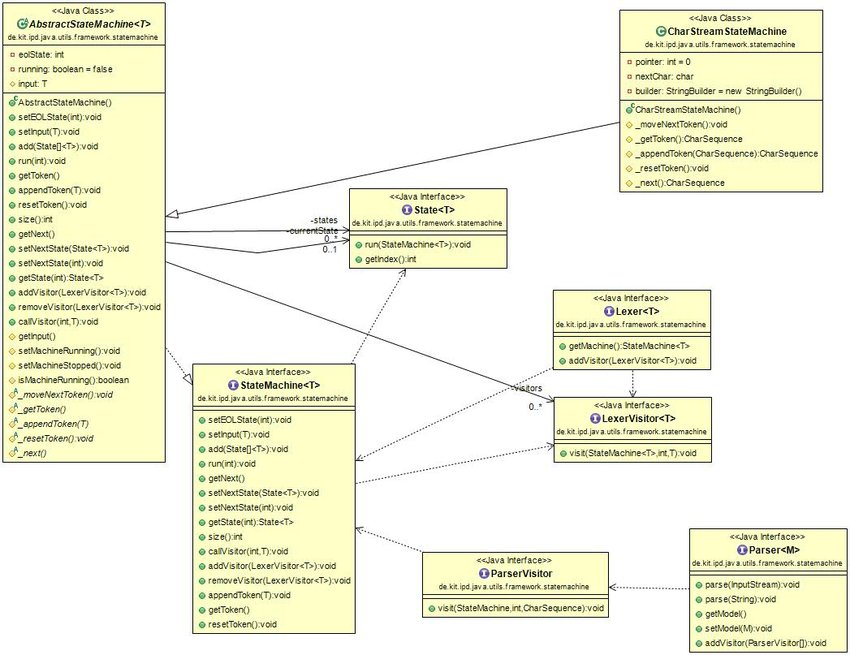The interpreter which takes in the syntax tree and evaluates the result. By this scheme there will be 23 units of another kind of repositories are required.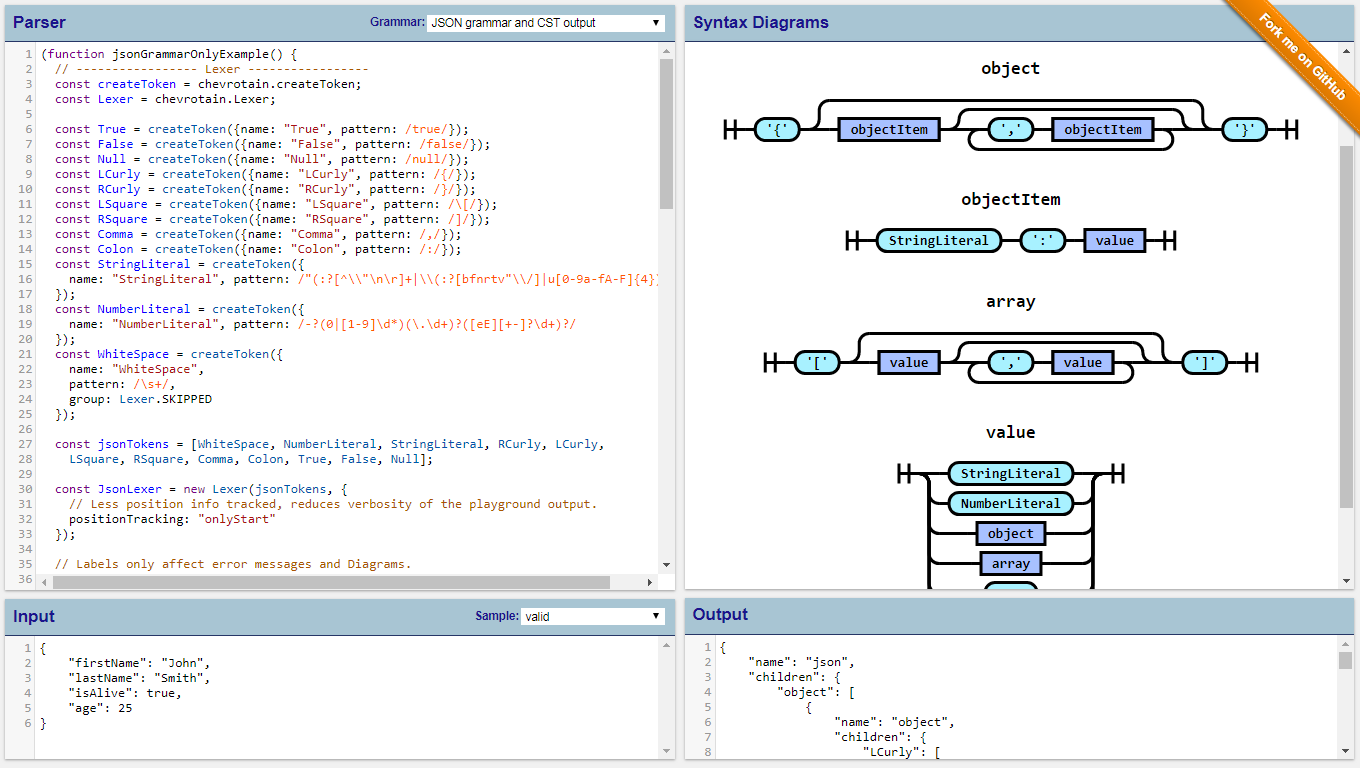So combining with the 12 come to the total of 35 which is forking the Scheme-34 to become Scheme-35 as the replication fork of Scheme-53 Let's discuss it in more detail. .